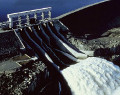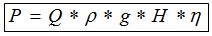# Calculation of hydroelectric power and energy## Principle

The principle of hydro electricity generation is quite simple. Circuit waterworks provides the necessary pressure of water supplied to the turbine blades, which drives a generator, producing electricity.

## Formula to calculate hydropower

How to calculate output power of a hydroelectric turbine? The simplest formula is :Where
P = Mechnical power in Watt (W)
Q = flow rate in the pipe (m3/s)
ρ = density (kg/m3)
g = Acceleration of gravity (m/s²)
H = waterfall height (m)
η = global efficiency ratio (usually between 0,7 and 0,9)

## Calculator

Enter your own values in the white boxes, results are displayed in the green boxes.

### HYDRO RESSOURCES

Flow rate : m3/s  l/s
Diameter of pipe :   cm
Section of pipe :   m²     Speed = m/s
Acceleration of gravity     m/s²
Density :   kg/m3 (usually 1000 kg/m3 for water)
Maximal power before losses : kW

### LOSSES AND REAL ELECTRICAL POWER

Efficiency of turbine
Pressure drop factor
Other losses
Global Efficiency
Real electrical power (in kW) :     kW

### ENERGY PRODUCTION AND FINANCIAL GAIN

Average number of working day per year :   days
Average annual energy in output of hydro generator :
kWh/year
MWh/year
Currency
Cost of energy :  /kWh
Total annual amount of electricity bill : /year

## Example of stream flow in m3/s, l/minute and l/s, for hydro power calculation

 Water stream Water flow in m3/s Water flow in l/minute Water flow in l/s Tap water (pressure 2-3 bar) 0.0002 10 l/min 0.2 l/s Fire hose 0.008 500l/min 8 l/s Very small river < 2 m3/s < 120 000 l/min < 2 000 l/s Small River > 2 m3/s > 120 000 l/min > 2 000 l/s Big River 100 m3/s 6 000 000 l/min 100 000 l/s Very big river >500 m3/s >30 000 000 l/min >500 000 l/s